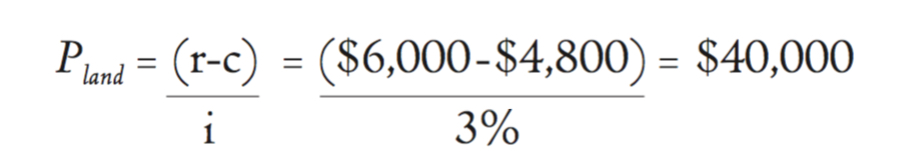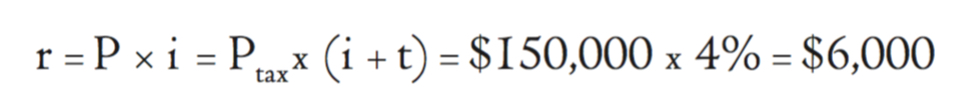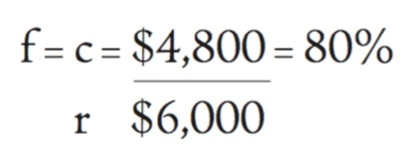# Appendix: The Math Behind the Science

Land prices are apt to change significantly with the introduction of community land contributions simply because land contributions prevent individuals and institutions from excessively profiting from land. While the following calculations offer us broad theoretical reference points, it isn’t necessary to comprehend the math in order to understand the theory behind land contributions; the math is only mentioned here for the interested reader.

If Pland is the purchase price of land under a land- contribution system, i the prevailing interest rate, r the land’s rental value, and c the contribution that is being collected, we use the following mathematical equation to estimate the new purchase price of land; for example, if i = 3%, r = \$6,000, and c = \$4,800, then:The rental value of land—its rent r—can be approximated using the property tax–free purchase price of land P and the prevailing interest rate i, or the purchase price of land with a property tax Ptax (we’ll assume a price of \$150,000) along with the interest rate i and the property tax rate t (we’ll assume a 1 percent property tax rate). This calculation is only an approximation, however, since purchase prices contain not only the capitalized rental value, but also include a speculative component as well (the speculative component will diminish the more land value is recovered):Land contributions of \$4,800 harness 80 percent of \$6,000, the rental value of land. The formula to calculate this fraction f is:A property valued at \$250,000 (with a land value of \$150,000, a building value of \$100,000, and a property tax rate of 1 percent) will be revalued at \$140,000 (with a land value of \$40,000 and a building value of \$100,000) with a land contribution of \$4,800 and no property tax—a 44 percent reduction.
Checkout